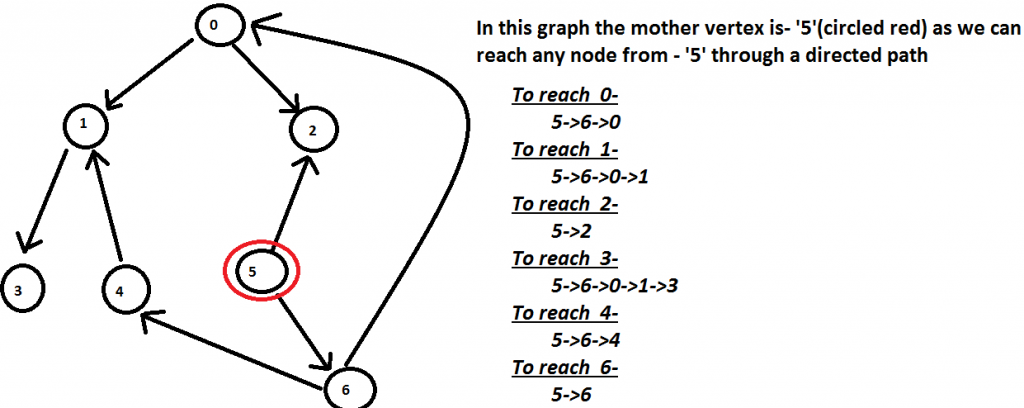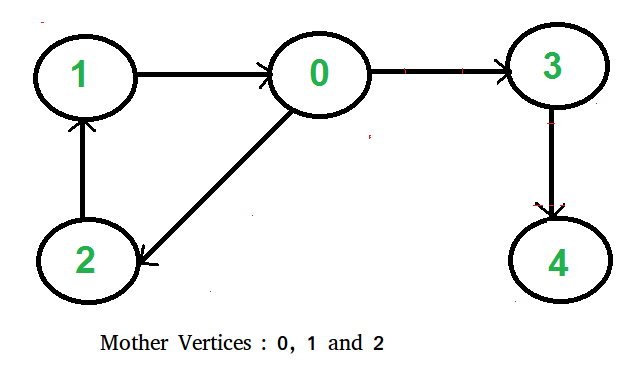# Find a Mother Vertex in a Graph

• Difficulty Level : Medium
• Last Updated : 30 Jun, 2022

What is a Mother Vertex?
A mother vertex in a graph G = (V, E) is a vertex v such that all other vertices in G can be reached by a path from v.

Example:

```Input  : Below Graph
Output : 5```There can be more than one mother vertices in a graph. We need to output anyone of them. For example, in the below graph, vertices 0, 1, and 2 are mother vertices.We strongly recommend you to minimize your browser and try this yourself first.

How to find mother vertex?

• Case 1:- Undirected Connected Graph : In this case, all the vertices are mother vertices as we can reach to all the other nodes in the graph.
• Case 2:- Undirected/Directed Disconnected Graph : In this case, there is no mother vertices as we cannot reach to all the other nodes in the graph.
• Case 3:- Directed Connected Graph : In this case, we have to find a vertex -v in the graph such that we can reach to all the other nodes in the graph through a directed path.

A Naive approach :
A trivial approach will be to perform a DFS/BFS on all the vertices and find whether we can reach all the vertices from that vertex. This approach takes O(V(E+V)) time, which is very inefficient for large graphs.

Can we do better?
We can find a mother vertex in O(V+E) time. The idea is based on Kosaraju’s Strongly Connected Component Algorithm. In a graph of strongly connected components, mother vertices are always vertices of source component in component graph. The idea is based on below fact.
If there exist mother vertex (or vertices), then one of the mother vertices is the last finished vertex in DFS. (Or a mother vertex has the maximum finish time in DFS traversal).
A vertex is said to be finished in DFS if a recursive call for its DFS is over, i.e., all descendants of the vertex have been visited.

How does the above idea work?
Let the last finished vertex be v. Basically, we need to prove that there cannot be an edge from another vertex u to v if u is not another mother vertex (Or there cannot exist a non-mother vertex u such that u-→v is an edge). There can be two possibilities.

1. Recursive DFS call is made for u before v. If an edge u-→v exists, then v must have finished before u because v is reachable through u and a vertex finishes after all its descendants.
2. Recursive DFS call is made for v before u. In this case also, if an edge u-→v exists, then either v must finish before u (which contradicts our assumption that v is finished at the end) OR u should be reachable from v (which means u is another mother vertex).

Algorithm :

1. Do DFS traversal of the given graph. While doing traversal keep track of last finished vertex ‘v’. This step takes O(V+E) time.
2. If there exist mother vertex (or vertices), then v must be one (or one of them). Check if v is a mother vertex by doing DFS/BFS from v. This step also takes O(V+E) time.

Below is the implementation of above algorithm.

## C++

 `// C++ program to find a mother vertex in O(V+E) time``#include ``using` `namespace` `std;` `class` `Graph``{``    ``int` `V;    ``// No. of vertices``    ``list<``int``> *adj;    ``// adjacency lists` `    ``// A recursive function to print DFS starting from v``    ``void` `DFSUtil(``int` `v, vector<``bool``> &visited);``public``:``    ``Graph(``int` `V);``    ``void` `addEdge(``int` `v, ``int` `w);``    ``int` `findMother();``};` `Graph::Graph(``int` `V)``{``    ``this``->V = V;``    ``adj = ``new` `list<``int``>[V];``}` `// A recursive function to print DFS starting from v``void` `Graph::DFSUtil(``int` `v, vector<``bool``> &visited)``{``    ``// Mark the current node as visited and print it``    ``visited[v] = ``true``;` `    ``// Recur for all the vertices adjacent to this vertex``    ``list<``int``>::iterator i;``    ``for` `(i = adj[v].begin(); i != adj[v].end(); ++i)``        ``if` `(!visited[*i])``            ``DFSUtil(*i, visited);``}` `void` `Graph::addEdge(``int` `v, ``int` `w)``{``    ``adj[v].push_back(w); ``// Add w to v’s list.``}` `// Returns a mother vertex if exists. Otherwise returns -1``int` `Graph::findMother()``{``    ``// visited[] is used for DFS. Initially all are``    ``// initialized as not visited``    ``vector <``bool``> visited(V, ``false``);` `    ``// To store last finished vertex (or mother vertex)``    ``int` `v = 0;` `    ``// Do a DFS traversal and find the last finished``    ``// vertex ``    ``for` `(``int` `i = 0; i < V; i++)``    ``{``        ``if` `(visited[i] == ``false``)``        ``{``            ``DFSUtil(i, visited);``            ``v = i;``        ``}``    ``}` `    ``// If there exist mother vertex (or vertices) in given``    ``// graph, then v must be one (or one of them)` `    ``// Now check if v is actually a mother vertex (or graph``    ``// has a mother vertex).  We basically check if every vertex``    ``// is reachable from v or not.` `    ``// Reset all values in visited[] as false and do``    ``// DFS beginning from v to check if all vertices are``    ``// reachable from it or not.``    ``fill(visited.begin(), visited.end(), ``false``);``    ``DFSUtil(v, visited);``    ``for` `(``int` `i=0; i

## Java

 `// Java program to find a mother``// vertex in O(V+E) time``import` `java.util.*;` `class` `GFG{``   ` `static` `void` `addEdge(``int` `u, ``int` `v,`` ``ArrayList> adj)``{``    ``adj.get(u).add(v);``}` `// A recursive function to print DFS starting from v``static` `void` `DFSUtil(ArrayList> g,``                    ``int` `v, ``boolean``[] visited)``{``    ``// Mark the current node as``    ``// visited and print it``    ``visited[v] = ``true``;``    ` `    ``// Recur for all the vertices``    ``// adjacent to this vertex``    ``for``(``int` `x : g.get(v))``    ``{``        ``if` `(!visited[x])``        ``{``            ``DFSUtil(g, x, visited);``        ``}``    ``}``}` `// Returns a mother vertex if exists.``// Otherwise returns -1``static` `int` `motherVertex(ArrayList>g,``                        ``int` `V)``{``    ` `    ``// visited[] is used for DFS. Initially``    ``// all are initialized as not visited``    ``boolean``[] visited = ``new` `boolean``[V];``    ` `    ``// To store last finished vertex``    ``// (or mother vertex)``    ``int` `v = -``1``;``    ` `    ``for``(``int` `i = ``0``; i < V; i++)``    ``{``        ``if` `(!visited[i])``        ``{``            ``DFSUtil(g, i, visited);``            ``v = i;``        ``}``    ``}``    ` `    ``// If there exist mother vertex (or vertices)``    ``// in given graph, then v must be one``    ``// (or one of them)``  ` `    ``// Now check if v is actually a mother``    ``// vertex (or graph has a mother vertex).``    ``// We basically check if every vertex``    ``// is reachable from v or not.``  ` `    ``// Reset all values in visited[] as false``    ``// and do DFS beginning from v to check``    ``// if all vertices are reachable from``    ``// it or not.``    ``boolean``[] check = ``new` `boolean``[V];``    ``DFSUtil(g, v, check);``    ``for``(``boolean` `val : check)``    ``{``        ``if` `(!val)``        ``{``            ``return` `-``1``;``        ``}``    ``}``    ``return` `v;``}` `// Driver code`` ``public` `static` `void` `main(String[] args)`` ``{``    ``int` `V = ``7``;``    ``int` `E = ``8``;``    ` `    ``ArrayList<``    ``ArrayList> adj = ``new` `ArrayList<``                                  ``ArrayList>();``    ``for``(``int` `i = ``0``; i < V; i++)``    ``{``        ``adj.add(``new` `ArrayList());``    ``}``    ``addEdge(``0``, ``1``,adj);``    ``addEdge(``0``, ``2``,adj);``    ``addEdge(``1``, ``3``,adj);``    ``addEdge(``4``, ``1``,adj);``    ``addEdge(``6``, ``4``,adj);``    ``addEdge(``5``, ``6``,adj);``    ``addEdge(``5``, ``2``,adj);``    ``addEdge(``6``, ``0``,adj);``    ` `    ``System.out.println(``"The mother vertex is "` `+``                        ``motherVertex(adj, V));``}``}` `// This code is contributed by Tanay Shah`

## Python3

 `# program to find a mother vertex in O(V+E) time``from` `collections ``import` `defaultdict` `# This class represents a directed graph using adjacency list``# representation``class` `Graph:` `    ``def` `__init__(``self``,vertices):``        ``self``.V ``=` `vertices ``#No. of vertices``        ``self``.graph ``=` `defaultdict(``list``) ``# default dictionary` `    ``# A recursive function to print DFS starting from v``    ``def` `DFSUtil(``self``, v, visited):` `        ``# Mark the current node as visited and print it``        ``visited[v] ``=` `True` `        ``# Recur for all the vertices adjacent to this vertex``        ``for` `i ``in` `self``.graph[v]:``            ``if` `visited[i] ``=``=` `False``:``                ``self``.DFSUtil(i, visited)` `    ``# Add w to the list of v``    ``def` `addEdge(``self``, v, w):``        ``self``.graph[v].append(w)` `    ``# Returns a mother vertex if exists. Otherwise returns -1``    ``def` `findMother(``self``):` `        ``# visited[] is used for DFS. Initially all are``        ``# initialized as not visited``        ``visited ``=``[``False``]``*``(``self``.V)` `        ``# To store last finished vertex (or mother vertex)``        ``v``=``0` `        ``# Do a DFS traversal and find the last finished``        ``# vertex``        ``for` `i ``in` `range``(``self``.V):``            ``if` `visited[i]``=``=``False``:``                ``self``.DFSUtil(i,visited)``                ``v ``=` `i` `        ``# If there exist mother vertex (or vertices) in given``        ``# graph, then v must be one (or one of them)` `        ``# Now check if v is actually a mother vertex (or graph``        ``# has a mother vertex). We basically check if every vertex``        ``# is reachable from v or not.` `        ``# Reset all values in visited[] as false and do``        ``# DFS beginning from v to check if all vertices are``        ``# reachable from it or not.``        ``visited ``=` `[``False``]``*``(``self``.V)``        ``self``.DFSUtil(v, visited)``        ``if` `any``(i ``=``=` `False` `for` `i ``in` `visited):``            ``return` `-``1``        ``else``:``            ``return` `v` `# Create a graph given in the above diagram``g ``=` `Graph(``7``)``g.addEdge(``0``, ``1``)``g.addEdge(``0``, ``2``)``g.addEdge(``1``, ``3``)``g.addEdge(``4``, ``1``)``g.addEdge(``6``, ``4``)``g.addEdge(``5``, ``6``)``g.addEdge(``5``, ``2``)``g.addEdge(``6``, ``0``)``print` `(``"A mother vertex is "` `+` `str``(g.findMother()))` `# This code is contributed by Neelam Yadav`

## C#

 `// C# program to find a mother``// vertex in O(V+E) time``using` `System;``using` `System.Collections.Generic;` `class` `GFG``{``   ` `static` `void` `addEdge(``int` `u, ``int` `v,`` ``List> adj)``{``    ``adj[u].Add(v);``}` `// A recursive function to print DFS starting from v``static` `void` `DFSUtil(List> g,``                    ``int` `v, ``bool``[] visited)``{``    ``// Mark the current node as``    ``// visited and print it``    ``visited[v] = ``true``;``    ` `    ``// Recur for all the vertices``    ``// adjacent to this vertex``    ``foreach``(``int` `x ``in` `g[v])``    ``{``        ``if` `(!visited[x])``        ``{``            ``DFSUtil(g, x, visited);``        ``}``    ``}``}` `// Returns a mother vertex if exists.``// Otherwise returns -1``static` `int` `motherVertex(List>g,``                        ``int` `V)``{``    ` `    ``// visited[] is used for DFS. Initially``    ``// all are initialized as not visited``    ``bool``[] visited = ``new` `bool``[V];``    ` `    ``// To store last finished vertex``    ``// (or mother vertex)``    ``int` `v = -1;  ``    ``for``(``int` `i = 0; i < V; i++)``    ``{``        ``if` `(!visited[i])``        ``{``            ``DFSUtil(g, i, visited);``            ``v = i;``        ``}``    ``}``    ` `    ``// If there exist mother vertex (or vertices)``    ``// in given graph, then v must be one``    ``// (or one of them)``  ` `    ``// Now check if v is actually a mother``    ``// vertex (or graph has a mother vertex).``    ``// We basically check if every vertex``    ``// is reachable from v or not.``  ` `    ``// Reset all values in visited[] as false``    ``// and do DFS beginning from v to check``    ``// if all vertices are reachable from``    ``// it or not.``    ``bool``[] check = ``new` `bool``[V];``    ``DFSUtil(g, v, check);``    ``foreach``(``bool` `val ``in` `check)``    ``{``        ``if` `(!val)``        ``{``            ``return` `-1;``        ``}``    ``}``    ``return` `v;``}` `// Driver code`` ``public` `static` `void` `Main(String[] args)`` ``{``    ``int` `V = 7;``    ``int` `E = 8;   ``    ``List<``    ``List<``int``>> adj = ``new` `List>();``    ``for``(``int` `i = 0; i < V; i++)``    ``{``        ``adj.Add(``new` `List<``int``>());``    ``}``    ``addEdge(0, 1,adj);``    ``addEdge(0, 2,adj);``    ``addEdge(1, 3,adj);``    ``addEdge(4, 1,adj);``    ``addEdge(6, 4,adj);``    ``addEdge(5, 6,adj);``    ``addEdge(5, 2,adj);``    ``addEdge(6, 0,adj);``    ` `    ``Console.WriteLine(``"The mother vertex is "` `+``                        ``motherVertex(adj, V));``}``}` `// This code is contributed by Rajput-Ji`

## Javascript

 ``

Output

`A mother vertex is 5`

Time Complexity : O(V + E)